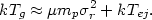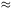5.3.3. Heating by ejection from galaxies

The presence of a nearly solar abundance of iron in the intracluster gas (Sections 4.3.2 and 5.2.3) suggests that a reasonable fraction of the gas may have come from stars in galaxies within the clusters. The gas ejected from galaxies is heated in two ways. First, the gas may have some energy when it is ejected. Letej be the total energy per unit mass of gas ejected from a galaxy in the rest frame of that galaxy, but not including the cluster gravitational potential, and define 3kTej / 2µ mpej. Second, the gas will initially be moving relative to the cluster center of mass at the galaxy's velocity. The ejected gas will collide with intracluster gas and thermalize its kinetic energy. On average this will give a temperature(5.27)

If the ejection energy can be ignored, the temperature is given by equation (5.14), in reasonable agreement with the observations (equation 4.10).

In a steady-state wind outflow from a galaxy, one expects kTejµ mp* 2, where*200 km/s is the velocity dispersion of stars within the galaxy. If the ejection temperature is near the lower limit given by this expression, then this form of heating will not be very important because*2 <<r2. However, the ejection temperature could be considerably higher. For example, supernovae within galaxies could both produce the heavy elements seen in cluster X-ray spectra and heat the gas in galaxies until it was ejected. Supernovae eject highly enriched gas at velocities of vSN104 km/s. The highly enriched, rapidly moving supernova ejecta would collide with the interstellar medium in a galaxy and heat the gas. If mSN is the mass of ejecta from a supernova and mej is the resulting total gas mass ejected from the galaxy, then Tej2 × 109 K(vSN / 104 km/s)2(MSN / Mej), which will be significant if the supernova ejecta are diluted by less than a factor of about 100.